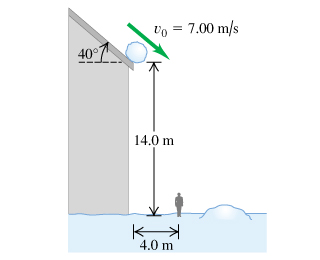# Problem: A snowball rolls off a barn roof that slopes downward at an angle of 40°. The edge of the roof is 14.0 m above the ground, and the snowball has a speed of 7.00 m/s { m m/s}as it rolls off the roof. Ignore air resistance. Assume the coordinate origin is at the point on the roof where the snowball rolls off and that the positive x direction is to the right and the positive y direction is upwards.(a) How far from the edge of the barn does the snowball strike the ground if it doesn't strike anything else while falling?(b) Draw y-t graphs for the motion in part A.(c) Draw x-t graphs for the motion in part A.(d) Draw vx-t graphs for the motion in part A.(e) Draw vy-t graphs for the motion in part A.(f) A man 1.9 m tall is standing 4.0 m from the edge of the barn. Will he be hit by the snowball?

81% (10 ratings)
###### Problem Details
A snowball rolls off a barn roof that slopes downward at an angle of 40°. The edge of the roof is 14.0 m above the ground, and the snowball has a speed of 7.00 m/s as it rolls off the roof. Ignore air resistance. Assume the coordinate origin is at the point on the roof where the snowball rolls off and that the positive x direction is to the right and the positive y direction is upwards.
(a) How far from the edge of the barn does the snowball strike the ground if it doesn't strike anything else while falling?
(b) Draw y-t graphs for the motion in part A.
(c) Draw x-t graphs for the motion in part A.
(d) Draw vx-t graphs for the motion in part A.
(e) Draw vy-t graphs for the motion in part A.
(f) A man 1.9 m tall is standing 4.0 m from the edge of the barn. Will he be hit by the snowball?Frequently Asked Questions

What scientific concept do you need to know in order to solve this problem?

Our tutors have indicated that to solve this problem you will need to apply the Projectile Motion: Horizontal & Negative Launch concept. If you need more Projectile Motion: Horizontal & Negative Launch practice, you can also practice Projectile Motion: Horizontal & Negative Launch practice problems.

How long does this problem take to solve?

Our expert Physics tutor, Julia took 9 minutes and 12 seconds to solve this problem. You can follow their steps in the video explanation above.

What professor is this problem relevant for?

Based on our data, we think this problem is relevant for Professor Sheets' class at RIT.